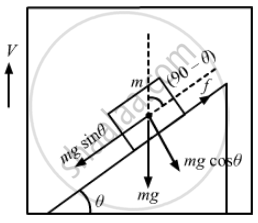Advertisement Remove all ads

# A Small Block of Mass M Is Kept on a Rough Inclined Surface of Inclination θ Fixed in an Elevator. the Elevator Goes up with a Uniform Velocity V And the Block Does Not Slide on the Wedge. - Physics

MCQ

A small block of mass m is kept on a rough inclined surface of inclination θ fixed in an elevator. the elevator goes up with a uniform velocity v and the block does not slide on the wedge. The work done by the force of friction on the block in time t will be

#### Options

• zero

• mgvt cos2θ

• mgvt sin2θ

• mgvt sin 2θ

Advertisement Remove all ads

#### Solution

mgvt sin2θ
Distance (d) travelled by the elevator in time t = vt
The block is not sliding on the wedge.
Then friction force (f) = mg sin $\theta$ Work done by the friction force on the block in time t is given by

$W = Fd\cos(90 - \theta)$

$\Rightarrow W = \text{ mg } \sin\theta \times d \times \cos(90 - \theta)$

$\Rightarrow W = \text{ mgd } \sin^2 \theta$

$\therefore W = \text{ mgvt } \sin^2 \theta$Is there an error in this question or solution?
Advertisement Remove all ads

#### APPEARS IN

HC Verma Class 11, 12 Concepts of Physics 1
Chapter 8 Work and Energy
MCQ | Q 8 | Page 131
Advertisement Remove all ads
Advertisement Remove all ads
Share
Notifications

View all notifications

Forgot password?